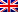# Combinatorial optimization

Contenus

## Combinatorial optimization

THE'combinatorial optimization, also called discrete optimization, is a branch of optimization in math applied and computer science, also related to operations research, algorithms and the theory of complexity. Combinatorial optimization consists in finding in a set a subset containing the “best solutions”.

Finding an optimal solution in a discrete and finite set is an easy problem in theory: you just have to try all the solutions, and compare their qualities to see the best. However, the combinatorial explosion of possible solutions of certain mathematical problems does not make it possible to obtain a solution in a "human" time.

Some combinatorial optimization problems can be solved (exactly) in polynomial time, for example by an algorithm of dynamic programming or by showing that the problem can be formulated as a linear optimization problem in real variables. In most cases, the problem is Np-hard and, to solve it, it is necessary to use suitable algorithms.

In practice, the physically acceptable complexity is often only polynomial. We are then satisfied with having a solution approximated at best, obtained by a heuristic or a meta-heuristic. It is important to note that some of these methods provide global optimals, the difference with the exact methods is the fact of not having a formal proof (mathematical or finality proof) of its global optimality.## Solution tree

That is E all the solutions to the problems. It is assumed to be discrete and finite. The enumeration of solutions is represented by tree. All elements of E are separated into not disjoint non-empty subset each containing a part of the set of solutions. For example, the set can be split into two in the problem of backpack whether or not we take an element xk in the bag.

The operation can be repeated for each subset until each set contains only one element. For the backpack example, each sub-assembly is separated until a decision is reached on the last element.

The root of the tree is E, the wires are the sub-assemblies etc. as shown in the following diagram:

# Solving techniques

## Exact

These methods give a guarantee to find the optimal solution for an instance of finite size in a limited time and to prove its optimality.

## Heuristic

When the complexity of a problem is exponential or presents a combinatorial explosion, the use of heuristics is recommended. This is a method that quickly provides a "good" solution to the problem. This approximate solution can then provide a starting point for using an exact method (such as the Northwest corner for the transport problem). All greedy and naive algorithms are heuristics.

It should be noted that heuristics are built to solve a given problem, and cannot be used in other conditions unlike meta-heuristics. Heuristics are evaluated according to three criteria:

1. Quality of the result: the heuristic is confronted with the optimal results for a set of values of the given problem (we speak of benchmark). The quality of the solution can either be a distance to the optimal solution, or a probability of reaching it.
2. Cost of heuristics: complexity in time and space.
3. Field of application: the field of admissibility of all the variables.

The heuristics for a given problem are numerous, so it is important to provide a fast one and provide "good" results.

## Meta-heuristic

Meta-heuristics have a higher level of abstraction than heuristics since it is a method that can adapt to a given problem. Thus, the methods can be applied to various problems (in the form of a black box) without modifying their operation. We also speak of generalist heuristics.

There are two kinds of meta-heuristics: population (To) or course (b). Most algorithms do not have a determined population, so it is possible to use the algorithm for a route or a population.

Both kinds work with the same four-step process:

1. Neighborhood : the neighborhood of a solution is a subset of solutions that can be reached by a transformation of the initial solution (by permutation, by extension, by mutation, by ejection, etc.).
2. Exploration : exploration consists of collecting data on the entire neighborhood.
3. Operation : the operation uses the information collected to define the “interesting” areas of the research area formed by the neighborhood.
4. Memory : the memory takes learning into account and makes it possible to determine the zones likely to have a global optimum. If the new solutions or the stopping criteria no longer allow the solution to be improved, the algorithm stops. Otherwise, return to step 1. Certain algorithms only work with stopping criteria, so we are talking about memoryless meta-heuristics.FRFRENES